Скачать презентацию A framework for eager encoding Daniel Kroening ETH

26b5af362129843444fa360973a1a705.ppt

• Количество слайдов: 13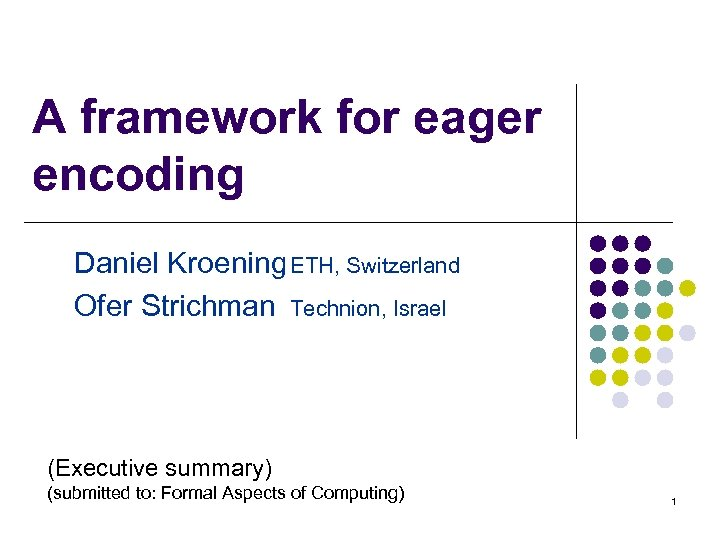A framework for eager encoding Daniel Kroening ETH, Switzerland Ofer Strichman Technion, Israel (Executive summary) (submitted to: Formal Aspects of Computing) 1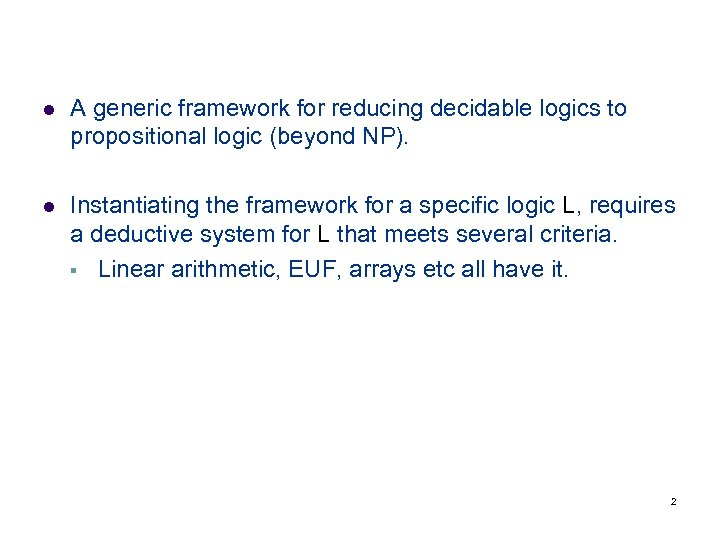l A generic framework for reducing decidable logics to propositional logic (beyond NP). l Instantiating the framework for a specific logic L, requires a deductive system for L that meets several criteria. § Linear arithmetic, EUF, arrays etc all have it. 2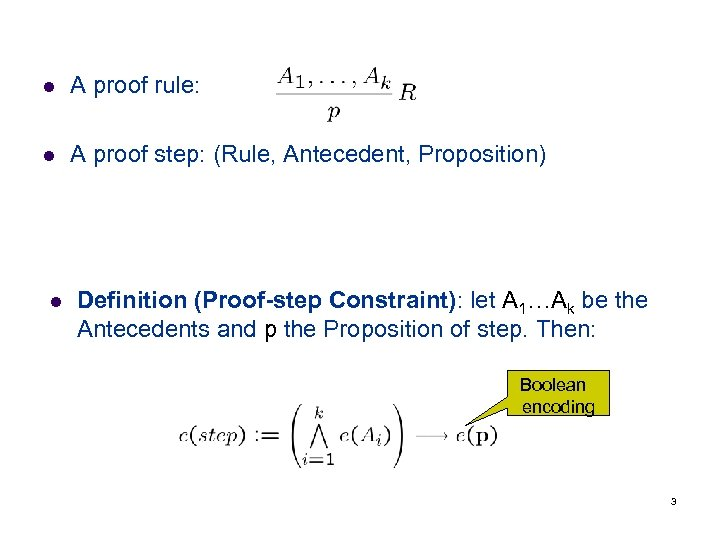l A proof rule: l A proof step: (Rule, Antecedent, Proposition) l Definition (Proof-step Constraint): let A 1…Ak be the Antecedents and p the Proposition of step. Then: Boolean encoding 3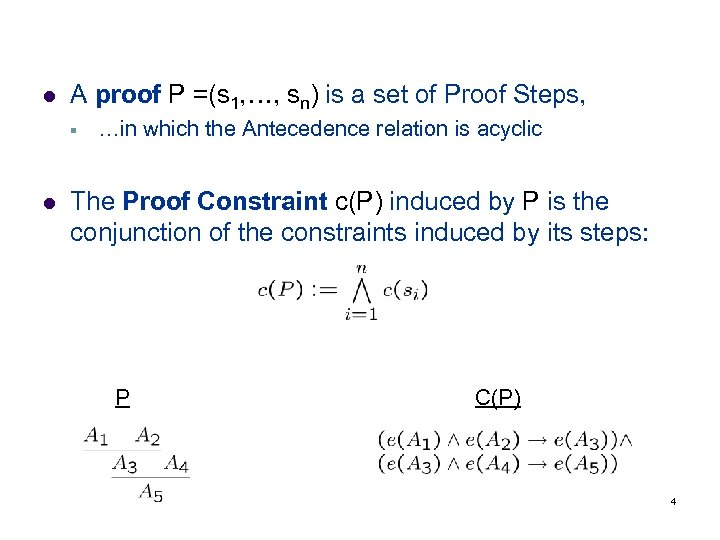l A proof P =(s 1, …, sn) is a set of Proof Steps, § l …in which the Antecedence relation is acyclic The Proof Constraint c(P) induced by P is the conjunction of the constraints induced by its steps: P C(P) 4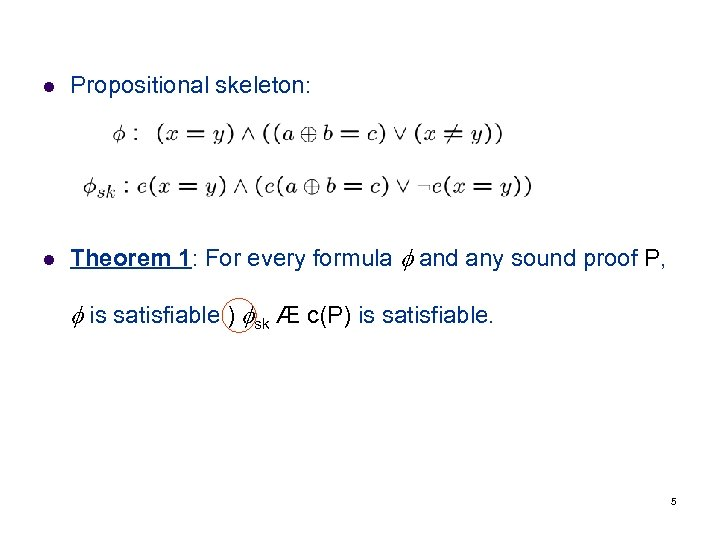l Propositional skeleton: l Theorem 1: For every formula and any sound proof P, is satisfiable ) sk Æ c(P) is satisfiable. 5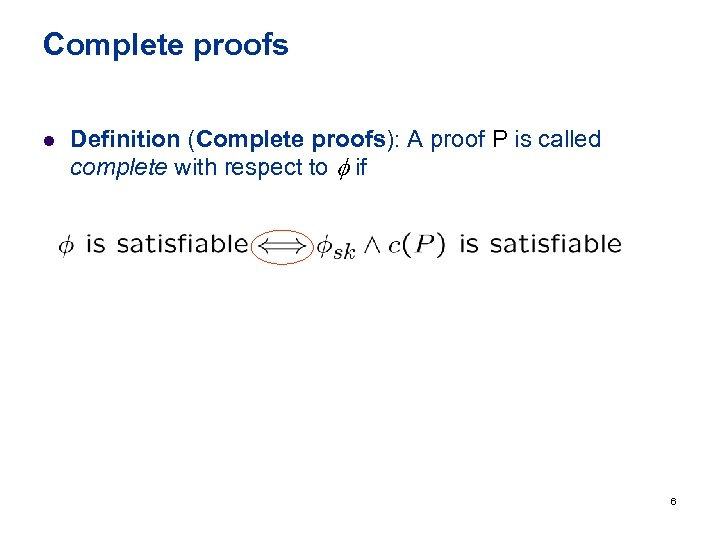Complete proofs l Definition (Complete proofs): A proof P is called complete with respect to if 6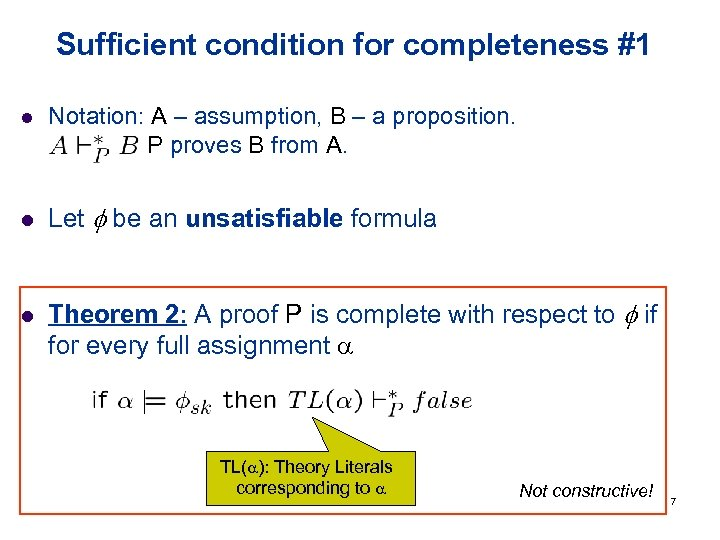Sufficient condition for completeness #1 l l l Notation: A – assumption, B – a proposition. denotes: P proves B from A. Let be an unsatisfiable formula Theorem 2: A proof P is complete with respect to if for every full assignment TL( ): Theory Literals corresponding to Not constructive! 7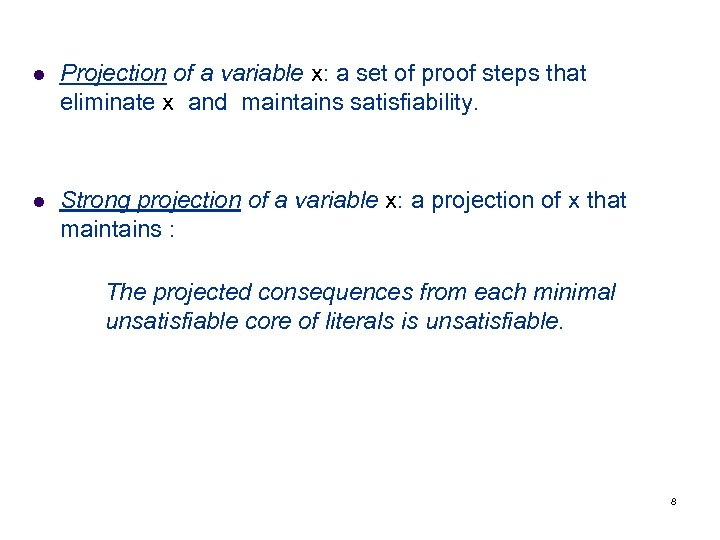l Projection of a variable x: a set of proof steps that eliminate x and maintains satisfiability. l Strong projection of a variable x: a projection of x that maintains : The projected consequences from each minimal unsatisfiable core of literals is unsatisfiable. 8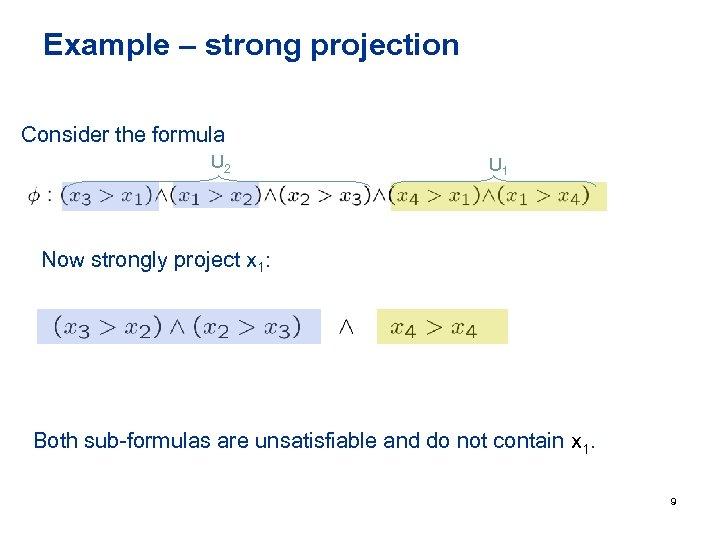Example – strong projection Consider the formula U 2 U 1 Now strongly project x 1: Both sub-formulas are unsatisfiable and do not contain x 1. 9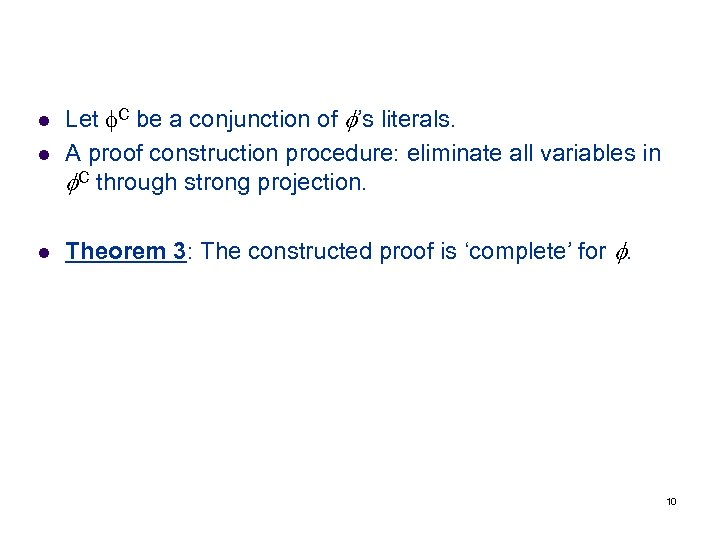l Let C be a conjunction of ’s literals. A proof construction procedure: eliminate all variables in C through strong projection. l Theorem 3: The constructed proof is ‘complete’ for . l 10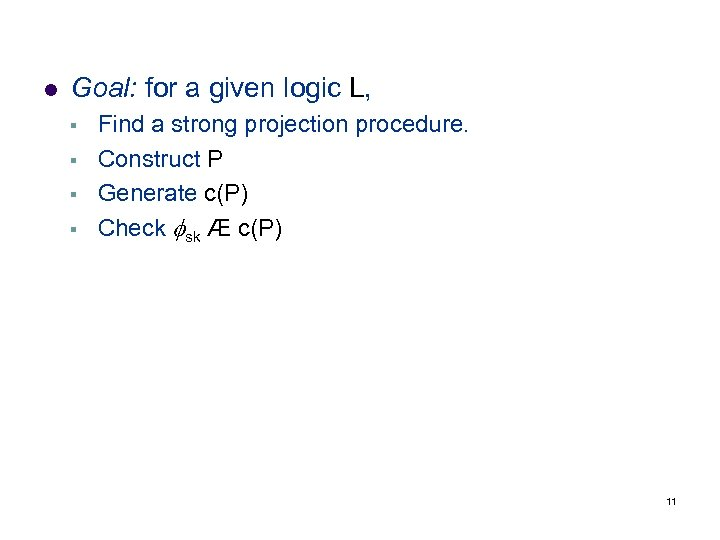l Goal: for a given logic L, § § Find a strong projection procedure. Construct P Generate c(P) Check sk Æ c(P) 11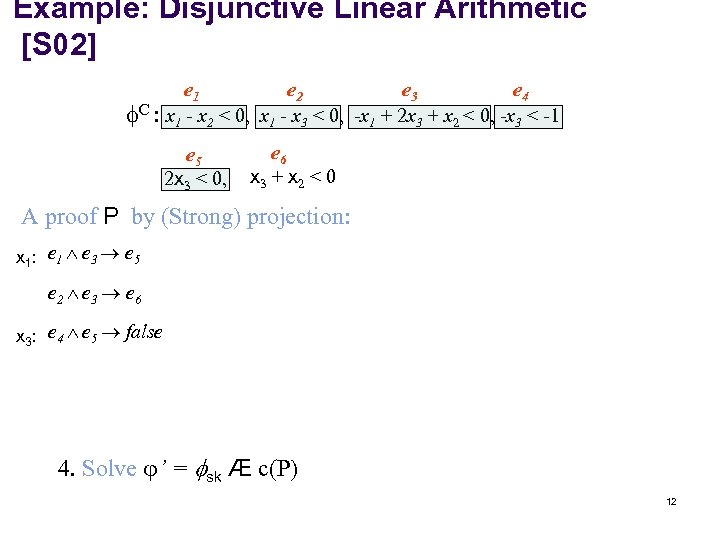Example: Disjunctive Linear Arithmetic [S 02] C : e 1 e 2 e 3 e 4 x 1 - x 2 < 0, x 1 - x 3 < 0, -x 1 + 2 x 3 + x 2 < 0, -x 3 < -1 e 5 2 x 3 < 0, e 6 x 3 + x 2 < 0 A proof P by (Strong) projection: x 1: e 1 e 3 e 5 e 2 e 3 e 6 x 3: e 4 e 5 false 4. Solve ’ = sk Æ c(P) 12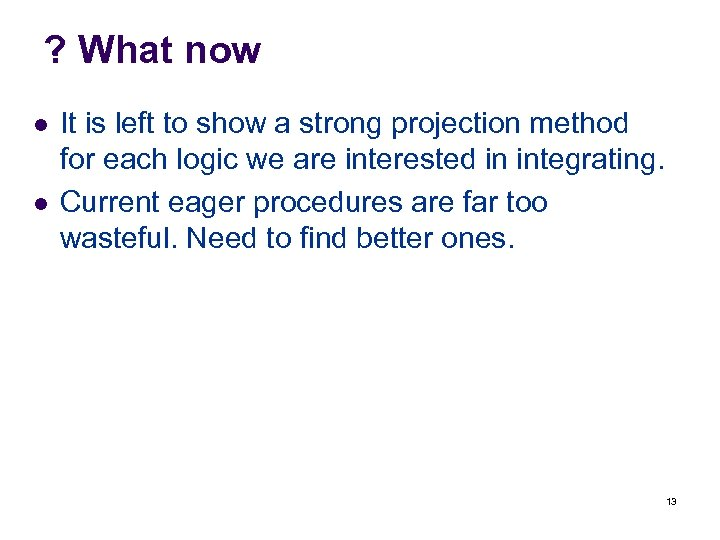? What now l l It is left to show a strong projection method for each logic we are interested in integrating. Current eager procedures are far too wasteful. Need to find better ones. 13